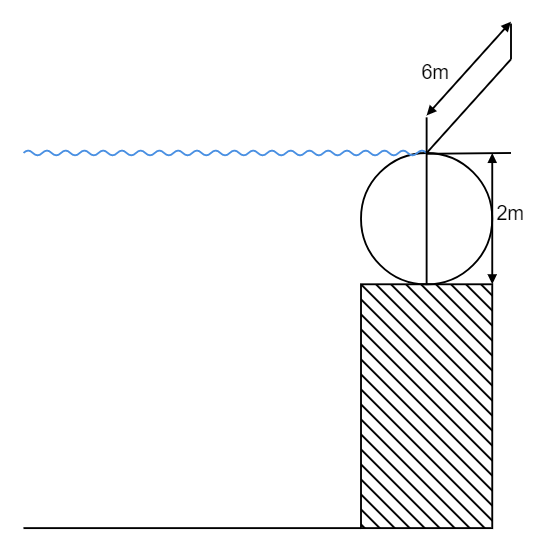Search
•APSEd

# Hydrostatic Force on a Submerged Surface | Center of Pressure Formula

Updated: Aug 2

Hydrostatic force is the resultant force acting on a submerged surface due to the pressure loading of the fluid into which the surface is submerged. The center of pressure is the point at which the hydrostatic force act.

## Hydrostatic Forces

As said, hydrostatic force is the resultant force acting on a submerged surface due to the pressure loading of the fluid into which the surface is submerged.

If the liquid is at rest, then there is no tangential force acting on the submerged surface and hence the total pressure will act normal to the surface in contact. Therefore, hydrostatic force is the resultant force acting on a submerged surface when the liquid is at rest.

### Center of Pressure

The location of the hydrostatic force is the center of pressure. The center of pressure is always below the center of gravity of the surface in contact.

## Hydrostatic Force Formula and Calculation

### Case 1: Horizontal Plane

In this case, the submerged surface is parallel to the surface of the water. The hydrostatic force can be simply calculated as the product of pressure exerted by the fluid and area of the surface.

Hydrostatic force on a horizontal plane,

Fh = (γ*h) * A

where,

γ - the unit weight of the fluid

h - depth at which the submerged plane is located

A - surface area of the submerged planeHydrostatic Force on Horizontal Plane

### Case 2: Vertical plane

In this case, the submerged surface is vertical i.e., perpendicular to the surface of the water. Integration is required to derive the hydrostatic force on a vertical plane formula.

Hydrostatic force on a vertical plane,

Fv = γ*A*h'

where,

γ - the unit weight of the fluid

A - total surface area

h' - the center of gravity of the surface

The center of pressure is given as,

hcp = h' + (Ig/Ah')

where,

Ig - the moment of inertia of the surface about its center of gravity

A - total surface area

h' - the center of gravity of the surface

The center of pressure (hcp) is always greater than the center of gravity (h') i.e., hcp > h'. Therefore, the center of pressure always lies below the center of gravity.Hydrostatic Force on Vertical Plane

### Case 3: Curved Profile Surface

In this case, the submerged surface has a curved profile, unlike the plain surfaces that we saw earlier. An example of such a surface is a sluice gate. In these cases, two forces are considered i.e., horizontal and vertical, and then the resultant force is found.

Horizontal force is determined by considering the vertical surface i.e., the vertical projection of the curved profile.

The horizontal force is given as,

Fh = γ * A * h'

where,

γ - the unit weight of the fluid

A - area of the vertical projection of the profile

h' - the center of gravity of the surface

Vertical force is the weight of liquid acting on the curved surface in contact.

The vertical force is given as,

Fv = γ * A contact * l = γ * Volume

where,

γ - the unit weight of the liquid

A contact - contact area of the profile

l - length of the profile

Resultant force, R = ((Fh^2) + (Fv^2))^(1/2)

Let us understand this through an example problem.

## Example Problem

Question: A spillway is shown below. Find the magnitude of the resultant force that is acting on the curved profile of the spillway.Solution:Horizontal force, Fh = γ * A * h'

Fh = 9.81 * (2*6) * (2/2)

Fh = 117.72 kN

Vertical force, Fv = γ * A contact * l

Fv = 9.81 * (((兀/4)*(d^2))/2) * 6

Fv = 92.457 kN

Resultant force, R = ((Fh^2) + (Fv^2))^(1/2)

R = 149.687 kN

## Practise Problem

We hope we had covered all the important aspects of hydrostatic forces on submerged bodies. This is an important topic preparing which could be really helpful in the GATE exam. We cover more such important topics on three topics per week basis at APSEd blog page. Do check them out!